Smartick is a fun way to learn math!May06

# Using Money to Help Learn Decimal Numbers

Today’s post deals with the day to day use of decimal numbers using money as an example (and to help you better understand it).

We are going to start with the basics, reviewing what decimal numbers are and how to read and write them.

You can use these resources to refresh your memory:

Now that you remember and have practiced with some of the exercises we showed you, we are going to apply this knowledge to real life.

Surely when you have gone shopping with your parents you have seen that most products have a price tag and, generally, this price is represented with a decimal number. Money is a very common way of using decimal numbers in our day to day life: dollars correspond to the whole part and cents, the decimal part.

Dollars consist of bills and coins:

• The dollar bills are:
• $1 •$2*
• $5 •$10
• $20 •$50
• $100 • The coins are: • 10¢ • 25¢ • 50¢* •$1*

*Although these bills and coins are minted, they are not commonly used.

In 1 dollar there are 100 cents and we write them after a period:

• 1 cent of a dollar is written $0.01 • 10 cents of a dollar is written$0.10
• 1 dollar and 1 cent is written $1.01 • 1 dollar and 10 cents is written$1.10

The same applies to larger numbers: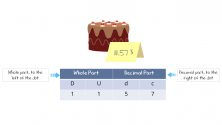In this case, we have eleven dollars and fifty-seven cents ($11.57). A dollar is a whole amount and is written to the left of the decimal. Cents are parts of a dollar and we write them to the right of the decimal. Take a look at the following image and look closely at the table with colored coins and the amount shown. There you can see a representation in the form of bills and coins: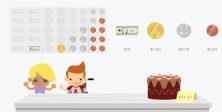As you can see, to form the whole part (eleven dollars), we use a$10 bill and a $1 coin. For the decimal part, (fifty-seven cents), we use five 10¢ coins and seven 1¢ coins. There are two ways to read this figure: 1. Eleven dollars and fifty-seven cents. 2. Eleven fifty-seven. #### We are going to practice with some exercises using decimal numbers How much money is there? We are going to express it with decimal numbers: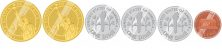In this first example, we have$2.31 (two dollars and thirty-one cents).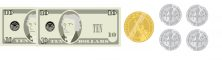In the second $21.40 (twenty-one dollars and forty cents).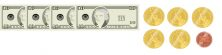Finally, we have$45.01 (forty-five dollars and one cent).

Now it’s your turn to practice every day. Each time you go shopping remember to look for the decimal numbers that are there, that way you can master this skill and start using it quickly.

If you would like to learn more topics in primary mathematics, register with Smartick and continue learning with us!